# NCERT Solutions for Class 4 Maths Table and Shares

NCERT solutions for Class 4 Mathematics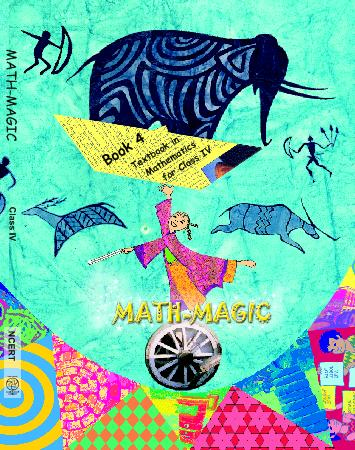## NCERT Solutions for Class 4 Maths Table and Shares

Chapter -11 Class 4 Maths Table and Shares

### 1. What are the ways in which the sunflower and marigold are planted?

Ans. 18 = 16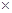3 So there are 6 rows with 3 plants each.

18 = 29 So there are 2 rows with 9 plants each.

### 2. Can you think of other ways to make a shelf to keep 30 jars?

Ans. The other ways to make a shelf to keep 30 jars can be as under:

30 = 103 So, there are 10 rows with 3 jars each.

30 = 310 So, there are 3 rows with 10 jars each.

30 = 56 So, there are 5 rows and 6 jars each.

30 = 65 So, there are 6 rows and 5 jars each.

### 3. Draw a shelf. Show how many jars you will keep in each row. How many rows are there?

Ans. A shelf to keep 30 jars is shown in the adjoining figure: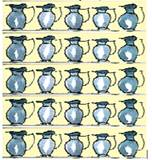Yes, my friends make it in different ways as under:

30 = 103 So, there are 10 rows with 3 jars each.

30 = 56 So, there are 5 rows with 6 jars each.

### 4. Help bunty to make the table of 7, using table of 4 and 3.

Ans. Table of 4: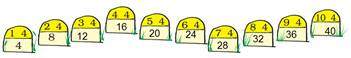Table of 3: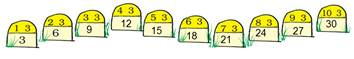Table of 7: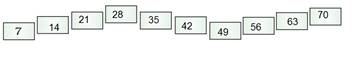In fact, we have added the two respective numbers in the lowest boxes of 4 and table of 3 to get the table of 7.

### 5. Which two tables will you use for writing the table of 12?

Ans. The tables of 4 and 8 can be used for writing the table of 12.

### 6.How many cats?

Ans.

 How many legs? 4 8 12 16 20 24 28 How many cats? 1 2 3 4 5 6 7

So, 28 legs mean 7 cats.

### 7. Billo has kept his chickens in a box. He counted 28 legs. How many chickens are there?

Ans.

 How many legs? 2 4 6 How many chickens? 1 2 3

So, 28 legs mean 14 chickens.

### 8. Leela has not gone to school for 21 days. For how many weeks was she away from school?

Ans.

 How many days? 7 14 21 How many weeks? 1 2 3

So, 21 days mean 3 weeks.

### 9. Jumping Animals:

(a) Count the jumps he takes to reach 27. So, he has taken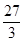= __ jumps.

Ans. (a) So, he has taken 9 jumps.

(b) He has taken 12 jumps if he is at 36.

Ans. (b) Because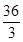= 12.

(c) If he is at 42, he has taken 14 jumps.

Ans. (c) Because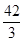= 14.

(d) In how many jumps does he reach 25?

Ans. He will reach 25 in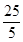= 5 jumps.

(e) He reaches 40 after taking 8 jumps.

Ans. (e) Because 85= 40.

(f) He needs 11 jumps to reach 55.

And. (f) Because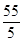= 11.

### 10. Sea Shells:

(a) How many shells are left now?

Ans. (a) 84 – 28 = 56 shells are left now.

(b) How many necklaces can Dhruv make from 112 shells?

Ans. (b) Dhruv can make 4 necklaces of 28 shells each from 112 shells.

(c) Are the shells enough for making necklaces for all his friends.

Ans. (c) Yes, the shells are enough for making necklaces for all his friends.

### 11. Try these:

(a) Kannu made a necklace of 17 sea-shellls. How many such necklaces can be made using 100 sea-shells?

Ans. (a) Let us do this problem by the process of repeated subtraction.

(1) 100 – 17 = 83

(2) 83 – 17 = 66

(3) 66 – 17 = 49

(4) 49 -17 = 32

(5) 32 – 17 = 15

Thus, Kannu can make 5 necklaces of 17 sea-shells using 100 sea-shells. He will also be left with 15 sea-shells as unused.

(b) One carton can hold 85 soaps. Shally wants to pack 338 soaps. How many cartons does the need for packing all of them?

Ans. (b) By the process of repeated subtraction, we have

(1) 338 – 85 = 253

(2) 253 – 85 = 168

(3) 168 – 85 = 83

(c) Manpreet wants 1500 sacks of cement for making a house. A truck carries 250 sacks at a time. How many trips will the truck make? A driver charges Rs 500 for a trip. How much will Manpreet pay the driver for all the trips?

Ans. (c) By the process of repeated subtraction, we have

(1) 1500 – 250 = 1250

(2) 1250 – 250 = 1000

(3) 1000 – 250 = 750

(4) 750 – 250 = 500

(5) 500 – 250 = 250

(6) 250 – 250 = 0

Thus, the truck will make 6 trips to carry 1500 sacks of cement.

Driver’s charge for 1 trip = Rs. 500

Therefore, driver’s charge for 6 trips = Rs. (5006) = Rs. 3000

Thus, the money paid by Manpreet to driver = Rs. 3000.

### 12. Gangu’s Sweets:

(a) Are the sweets in the tray enough to pack 23 small boxes?

Ans. (a) Laddoos needed for 23 small boxes at the rate of 4 per pack = 423 = 92. Because 92 > 80, so the sweets in the tray are not enough.

(b) How many more sweets are needed?

Ans. (b) Number of more sweets needed = 92 – 80 = 12.

(c) Gangu also has a bigger box in which he pack 12 laddoos. How many boxes does he used he need for packing 60 laddoos?

Ans. (c) Number of boxes needed for packing 60 laddoos at the rate of 12 per pack =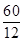= 5.

### 13. Neelu brought 15 storybooks to her class. Today 45 students are present. How many children will need to share one book?

Ans. Number of students = 45

Number of story books = 15

Number of children sharing one book =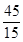= 3

Thus, 3 children will need to share one book.

### 14. A family of 8 people needs 60 kg wheat for a month. How much wheat does this family need for a week?

Ans. Wheat needed by a family for a month =60 kg

Therefore, wheat needed by a family for a week =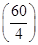= 15 kg.

### 15. Razia wants change for Rs. 500. How many notes will she get if she wants in return.

Ans. (1) Number of notes required =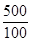= 5

(2) Number of notes required =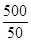= 10

(3) Number of notes required =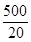= 25

(4) Number of notes required =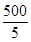= 100

Class 4 Maths Table and Shares

### 16. You have to distribute 72 tomatoes equally in baskets. How many tomatoes will there be in each?

Ans. Number of tomatoes = 72

Number of tomatoes distributed equally per basket =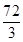= 24.

### 17. There are 350 bricks in a hand-cart. Binod found the weight of a brick to be 2 kg. What will be the weight of all the blocks?

Ans. Number of brick in a hand-cart = 350

Weight of 1 brick = 2 kg

Total weight of 350 bricks = (3502) kg = 700 kg

### 18. How will Lokesh distribute the rest of the money? Complete it.

Ans. Lokesh will distribute the rest of the money, that is, Rs. 15 among 5 by finding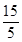, which is 3.

Or, it can be completed as under: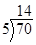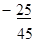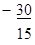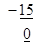Lokesh distributed Rs. 3 more. Now nothing is left. And all the money is divided equally.

So, each child gets 5 + 6+ 3 = 14 rupees.

Since, 145 = 70, so answer is correct.

Class 4 Maths Table and Shares

### 19. Now use your own method to divide Rs. 70 equally among 5 people. If you want you can start by giving Rs. 2 to each. Or you can even start with Rs. 11 to each.

First, I give Rs. 11 to each.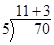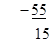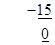I have distributed 115 = 55 rupees. Next, I give Rs. 3 more to everyone. I have distributed 53 – 15 rupees. Now nothing is left. And all the money is divided equally. So, each child gets 11+ 3= 14 rupees.

Ans. We cannot start with Rs. 15 as Rs. 155 = Rs. 75, which is more than Rs. 70.

### 21.Try doing these:

(a)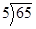Ans. (a)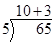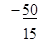(b)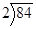Ans. (b)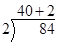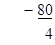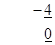(c)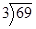Ans. (c)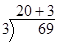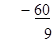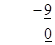(d)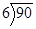Ans. (d)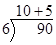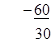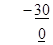(e)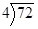Ans. (e)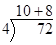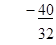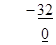(f)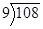Ans. (f)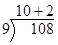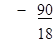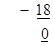(g)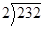Ans. (g)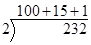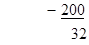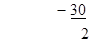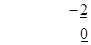(h)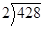Ans. (h)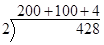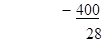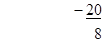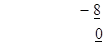(i) Meera made 204 candles to sell in the market. She makes packets of 6. How many packets will she make? If she packs them in packets of 12 then how many packets will she make?

Ans. (i) Number of candles = 204

Number of packets at the rate of 6 packets =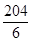= 34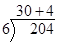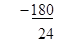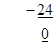Thus, the required number of packets = 34.

Number of packet @ 12 per packet =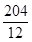= 17.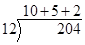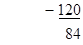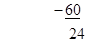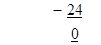Thus, the required number of packet = 17.

(j) On sports day 161 children are in the school playground. They are standing in 7 equal rows. How many children are there in each row?

Ans. (i) Number of children = 161

They are standing in 7 equal rows.

Number of children per row =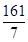=23

Thus, there are 23 children standing in each row.

Class 4 Maths Table and Shares

### 22. Now you look at the other picture and make questions like Srishti.

(a) There are 8 packets of rachis. Each packet has 7 rakhis in it.

Ans. (a) My question: How many rakhis are there in all?

Number of rakhis = 87 =56

(b) There are 10 packets of sugar. Saurabha paid 110 rupees for all the packets.

Ans. (b) My question: What is the cost of 1 packet of sugar?

Cost of one packet of sugar =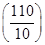rupees = 11 rupees

(c) There are 35 students in 7 rows. Each row has the same number of students.

Ans. (c) My question: How many students are there in each row?

Number of students in each row =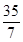= 5 students

(d) Hari, Seema, Chinku and Lakshmi are going to Guwahati. The cost of one rail ticket is Rs. 62.

Ans. (d) My question: How much is the cost of four rail tickets?

Cost of 4 rail tickets = Rs. 624 = Rs. 248.

(e) One metre of cloth cost Rs. 20. Lalbiak bought some cloth and paid Rs. 140.

Ans. (e) My question: How many metres of cloth was bought for Rs. 140?

Length of cloth bought for R. 140=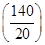m = 7 m.

## NCERT solutions for Class 4 Mathematics Chapter 11 Table and Shares

Building with BricksView Solutions
Long and ShortView Solutions
A trip to BhopalView Solutions
Tick Tick TickView Solutions
The Way the World LooksView Solutions
The Junk SellerView Solutions
Jugs and MugsView Solutions
Carts and WheelsView Solutions
Halves and QuartersView Solutions
Play With PatternsView Solutions
Table and SharesView Solutions
How Heavy How LightView Solutions
Fields And FencesView Solutions
Smart ChartsView Solutions

NCERT SolutionsClass 4 Maths Table and Shares PDF (Download) Free from myCBSEguide app and myCBSEguide website. Ncert solution class 4 Maths includes text book solutions from Class 4 Maths Book . NCERT Solutions for CBSE Class  Maths have total 14 chapters. 4 Maths NCERT Solutions in PDF for free Download on our website. Ncert Maths class 4 solutions PDF and Maths ncert class 4 PDF solutions with latest modifications and as per the latest CBSE syllabus are only available in myCBSEguide.

## CBSE app for Students

To download NCERT Solutions for Class 4 Maths, EVS Hindi English, Maths Science do check myCBSEguide app or website. myCBSEguide provides sample papers with solution, test papers for chapter-wise practice, NCERT solutions, NCERT Exemplar solutions, quick revision notes for ready reference, CBSE guess papers and CBSE important question papers. Sample Paper all are made available through the best app for CBSE students and myCBSEguide website.### Test Generator

Create question paper PDF and online tests with your own name & logo in minutes.### myCBSEguide

Question Bank, Mock Tests, Exam Papers, NCERT Solutions, Sample Papers, Notes

### 7 thoughts on “NCERT Solutions for Class 4 Maths Table and Shares”

1. This is very very very much greater

2. 22.question is wrong because each packet has 6 rakhis not 7 rakhis